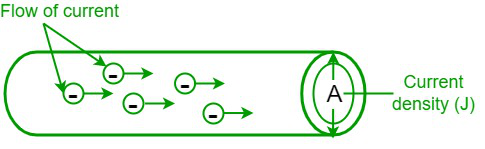# How to Calculate Current Density?

• Last Updated : 01 Jul, 2022

Electricity has become an essential part of our modern lives. We need electricity to light up the dark room and run all our appliances like fans, mixers, air conditioners, televisions, etc. In fact, we can not imagine a world without electricity. Electricity is referred to as a form of energy that results due to the flow of electrons. There are two kinds of electricity, namely, static electricity and current electricity. As the use of electricity is increasing nowadays, it is produced in large quantities and stored. Current is defined as the rate of charge flow from negative points to positive points in an electric circuit. It is measured in terms of amperes (A) and is denoted by the letter (I). There are two kinds of current, namely, alternating current and direct current.

### Current density

In physics, current density, or the electric current density, is related to electromagnetism and defined as the measure of current flowing through a unit value of the area of the cross-section. Electric current (I) is a macroscopic entity, and we define a corresponding microscopic entity called current density. Current density has both magnitude and direction since it is a vector quantity. In the case of a steady current, the flow of current is uniform throughout the conductor even if the area of cross-section differs. With the help of the current density, we can calculate the amount of current flowing in a specified portion of the conductor. It is represented by the letter “J” and its SI unit is A/m2.

Let us consider a conducting wire and connect both ends of the wire to a battery. Now an electric field will be produced, which results in the flow of electrons through the conductor. Now, if σ is the electrical conductivity of the conducting wire, then the current density is directionally proportional to the electric field.

Current density (J) = σ E

Where σ is the electrical conductivity,

E is the electric field.The formula for current density is given as,

Current density (J) = I/A

Where “I” is the current flowing the conductor,

“A” is the cross-sectional area of the conductor.

The dimensional formula of the current density is M0L-2T0I1 where M is mass, L is length, T is time, and I is current.

### Solved Problems

Problem 1: Calculate the current density if a current of 60 A is flowing through a copper wire with a cross-sectional area of 20 m2.

Solution:

Given data,

Current flowing through the wire (I) = 60 A

The cross-sectional area of the wire (A) = 20 m2

We know that,

Current density (J) = I/A

J = (60)/(20)

J = 3 A/m2

Hence, the current density is 3 A/m2.

Problem 2: Determine the current density if a current of 5A is flowing through a copper wire whose diameter is 3 mm.

Solution:

Given data,

Current flowing through the wire (I) = 5A

Diameter of the wire = 3mm

⇒ Radius = 3/2 = 1.5 mm = 0.0015 m

Area of the cross-section of the wire = πr2

A = 3.14 × (0.0015)2

A = 0.000007065 m2

We know that,

Current density (J) = I/A

J = 5/0.000007065

J = 7.07 × 105 A/m2

Thus, the current density is 7.07 × 105 A/m2.

Problem 3: Find the current flowing through the metal wire if its cross-sectional area is 15 m2 and the current density is 5 A/m2.

Solution:

Given data,

Cross-sectional area (A) = 15 m2

Current density (J) = 5 A/m2

We know that,

Current density (J) = I/A

5 = I/15

I= 5 × 15 = 75 A

Hence, the current flowing through the wire is 75 A.

Problem 4: Find the cross-sectional area of the wire if the current flowing through the wire is 4 mA and its current density is 0.25 A/m2.

Solution:

Given data,

Current flowing the wire (I) = 4 mA = 0.004 A

Current density (J) = 0.25 A/m2

We know that,

Current density (J) = I/A

0.25 = 0.004/A

A = 0.004/0.25

A = 0.016 m2 = 16 mm2

Hence, the cross-sectional area of the wire is 16 mm2.

Problem 5: Determine the current density if a current of 85 A is flowing through a conductor with a cross-sectional area of 17 m2.

Solution:

Given data,

Current flowing through the wire (I) = 85 A

The cross-sectional area of the wire (A) = 17 m2

We know that,

Current density (J) = I/A

J = 85/17

J = 5 A/m2

Therefore, the current density is 5 A/m2.

Problem 6: What is the definition of current density and its SI unit of measurement?

Solution:

In physics, current density, or the electric current density, is defined as the measure of current flowing through a unit value of the area of the cross-section. It is represented by the letter “J” and its S.I unit is ampere per square meters (A/m2).

My Personal Notes arrow_drop_up
Recommended Articles
Page :